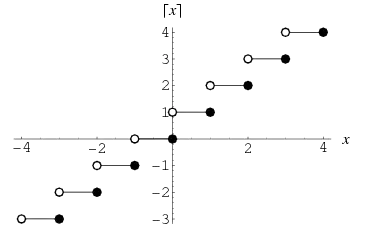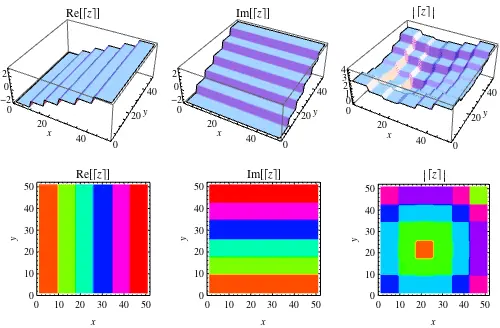Ceiling FunctionThe function  which gives the smallest integer , shown as the thick curve in the above plot. Schroeder (1991) calls the ceiling function symbols the "gallows" because of the similarity in appearance to the structure used for hangings. The name and symbol for the ceiling function were coined by K. E. Iverson (Graham et al. 1994).Min    Max

Re

Register for Unlimited Interactive Examples >>

Im

The ceiling function is implemented in Mathematica as Ceiling[z], where it is generalized to complex values of  as illustrated above.

Although some authors used the symbol  to denote the ceiling function (by analogy with the older notation  for the floor function), this practice is strongly discouraged (Graham et al. 1994, p. 67). Also strongly discouraged is the use of the symbol  to denote the ceiling function (e.g., Harary 1994, pp. 91, 93, and 118-119), since this same symbol is more commonly used to denote the fractional part of .

Since usage concerning fractional part/value and integer part/value can be confusing, the following table gives a summary of names and notations used. Here, S&O indicates Spanier and Oldham (1987).

notation    name    S&O    Graham et al.    Mathematica

ceiling function    --    ceiling, least integer    Ceiling[x]

congruence    --    --    Mod[m, n]

floor function    floor, greatest integer, integer part    Floor[x]

fractional value    fractional part or     SawtoothWave[x]

fractional part    no name    FractionalPart[x]

integer part    no name    IntegerPart[x]

nearest integer function    --    --    Round[x]

quotient    --    --    Quotient[m, n]

SEE ALSO: Floor Function, Fractional Part, Integer Part, Mills' Constant, Mod, Nearest Integer Function, Power Ceilings, Quotient, Staircase Function

RELATED WOLFRAM SITES: http://functions.wolfram.com/IntegerFunctions/Ceiling/

REFERENCES:

Croft, H. T.; Falconer, K. J.; and Guy, R. K. Unsolved Problems in Geometry. New York: Springer-Verlag, p. 2, 1991.

Graham, R. L.; Knuth, D. E.; and Patashnik, O. "Integer Functions." Ch. 3 in Concrete Mathematics: A Foundation for Computer Science, 2nd ed. Reading, MA: Addison-Wesley, pp. 67-101, 1994.

Iverson, K. E. A Programming Language. New York: Wiley, p. 12, 1962.

Schroeder, M. Fractals, Chaos, Power Laws: Minutes from an Infinite Paradise. New York: W. H. Freeman, p. 57, 1991.

Spanier, J.; Myland, J.; and Oldham, K. B. An Atlas of Functions, 2nd ed. Washington, DC: Hemisphere, 1987.